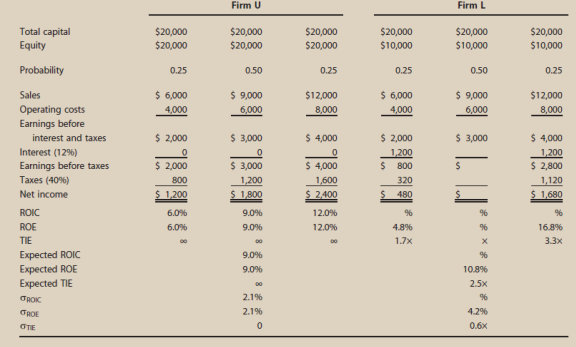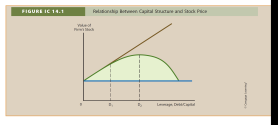Chapter 14, Problem 15ICFundamentals of Financial Manageme...

14th Edition
Eugene F. Brigham + 1 other
ISBN: 9781285867977

Solutions

Chapter
SectionFundamentals of Financial Manageme...

14th Edition
Eugene F. Brigham + 1 other
ISBN: 9781285867977
Textbook Problem

OPTIMAL CAPITAL STRUCTURE Assume that you have just been hired as business manager of Campus Deli (CD), which is located adjacent to the campus. Sales were $1,100,000 last year, variable costs were 60% of sales, and fixed costs were$40,000. Therefore, EBIT totaled $400,000. Because the university’s enrollment is capped, EBIT is expected to be constant over time. Because no expansion capital is required, CD distributes all earnings as dividends. Invested capital is$2 million, and 80,000 shares are outstanding. The management group owns about 50% of the stock, which is traded in the over-the counter market.CD currently has no debt—it is an all-equity firm—and its 80,000 shares outstanding sell at a price of $25 per share, which is also the book value. The firm’s federal-plus-state tax rate is 40%. On the basis of statements made in your finance text, you believe that CD’s shareholders would be better off if some debt financing were used. When you suggested this to your new boss, she encouraged you to pursue the idea but to provide support for the suggestion.In today’s market, the risk-free rate, rRF, is 6%, and the market risk premium, RPM, is 6%. CD’s unlevered beta, bU, is 1 0. CD currently has no debt, so its cost of equity (and WACC) is 12%. If the firm was recapitalized, debt would be issued and the borrowed funds would be used to repurchase stock. Stockholders, in turn, would use funds provided by the repurchase to buy equities in other fast-food companies similar to CD. You plan to complete your report by asking and then answering the following questions. a. 1. What is business risk? What factors influence a firm’s business risk? 2. What is operating leverage, and how does it affect a firm’s business risk? 3. What is the firm’s return on invested capital (ROIC)? b. 1. What do the terms financial leverage and financial risk mean? 2. How does financial risk differ from business risk? c. To develop an example that can be presented to CD’s management as an illustration, consider two hypothetical firms: Firm U with zero debt financing and Firm L with$ , of 12% debt. Both firms have $20,000 in invested capital and a 40% federal-plus-state tax rate, and they have the following EBIT probability distribution for next year: Probability EBIT 025$2,000 0.50 3,000 025 4,000 1. Complete the partial income statements and the firms’ ratios in Table IC 14.1.2. Be prepared to discuss each entry in the table and to explain how this example illustrates the effect of financial leverage on expected rate of return and risk.d. After speaking with a local investment banker, you obtain the following estimates of the cost of debt at different debt levels (in thousands of dollars): Amount Borrowed Debt/Capital Ratio D/E Ratio Bond Rating r<j $0 0 0 — — 250 0.125 0.1429 AA 8.0% 500 0.250 03333 A 9.0 750 0375 0.6000 BBB 113 1,000 0.500 1.0000 BB 14.0 Now consider the optimal capital structure for CD.1. To begin, define the terms optimal capital structure and target capital structure.2. Why does CD’s bond rating and cost of debt depend on the amount of money borrowed?3. Assume that shares could be repurchased at the current market price of$25 per share. Calculate CD’s expected EPS and TIE at debt levels of $0,$250,000, $500,000,$750,000, and $1,000,000. How many shares would remain after recapitalization under each scenario?4. Using the Hamada equation, what is the cost of equity if CD recapitalizes with$250,000 of debt? $500,000?$750,000? $1,000,000?5. Considering only the levels of debt discussed, what is the capital structure that minimizes CD’s WACC?6. What would be the new stock price if CD recapitalizes with$250,000 of debt? $500,000?$750,000? \$1,000,000? Recall that the payout ratio is 100%, so g 0.7. Is EPS maximized at the debt level that maximizes share price? Why or why not?8. Considering only the levels of debt discussed, what is CD’s optimal capital structure?9. What is the WACC at the optimal capital structure? d. Suppose you discovered that CD had more business risk than you originally estimated. Describe how this would affect the analysis. How would the analysis be affected if the firm had less business risk than originally estimated? e. What are some factors a manager should consider when establishing his or her firm’s target capital structure? f. Put labels on Figure IC 14.1 and then discuss the graph as you might use it to explain to your boss why CD might want to use some debt. g. How does the existence of asymmetric information and signaling affect capital structure?a.1.

Summary Introduction

To determine: Business risk and factors which influence firm’s business risks.

Introduction:

Optimal Capital Structure:

The optimum mix of debt and equity in the capital structure of the company is known as the optimum capital budget. The optimum capital budget is also known as the optimum capital structure.

Business risks are elements which have an adverse effect on the profitability and performance of the business.

Explanation

Given below are the factors which influence the firm’s business risks:

Business risks differ from business to business and from department to department.

• Fluctuation in the demand of the product
• Fluctuation in the selling price of the product...

a2.

Summary Introduction

To determine: Operating leverage and its effect on business risk of a firm.

Introduction:

Operating Leverage:

Operating leverage is the proportion of the fixed cost of the business in comparison to the total cost of the business.

a3.

Summary Introduction

To explain: The return on invested capital of the firm.

Introduction:

Return on Invested Capital:

It indicates the capability of the firm in generating revenue against the sum invested.

b1.

Summary Introduction

To explain: Financial leverage and financial risks.

Introduction:

Financial Leverage:

Financial leverage indicates the extent of fixed income securities is utilized to purchase additional assets.

Financial Risks:

Financial risks are generally referred to risks that arise due to financial decisions.

b2.

Summary Introduction

To explain: Difference between financial risk and business risk.

Introduction:

Financial Risks:

Financial risks are generally referred to risks that arise due to financial decisions.

Business risks are elements which have an adverse effect on the profitability and performance of the business.

c1.

Summary Introduction

To prepare: Partial income statement and the firm’s ratios.

C2.

Summary Introduction

To identify: The effect of financial leverage on the expected rate of return and risk.

d1.

Summary Introduction

To discuss: The meaning of Optimal Capital Structure and Target Capital Structure.

Introduction:

Optimal Capital Structure:

The optimum mix of debt and equity in the capital structure of the company is known as the optimum capital budget. The optimum capital budget is also known as the optimum capital structure.

Target Capital Structure:

The optimal capital structured required by the firm in order to maintain firm’s stock price.

d2.

Summary Introduction

To determine: Dependence of bond rating and cost of debt on the borrowed money.

Introduction:

Bond Rating:

The bond rating is the classification of a different class of bonds depending on factors such as creditworthiness of bonds, its ability to pay interest and principal timely.

Cost of Debt:

Cost of debt represents the percentage of amount company pays as interest on the borrowed funds.

d3.

Summary Introduction

To compute: C Company’s EPS and TIE.

d4.

Summary Introduction

To compute: Cost of equity.

Introduction:

Hamada equation is a tool to measure the effect of an increase in debt on the cost of equity. It is used to distinguish the financial risk of a levered firm from its business risk.

d5.

Summary Introduction

To compute: Capital structure that minimizes C Company’s WACC.

d6.

Summary Introduction

To compute: New stock price.

d7.

Summary Introduction

To identify: Whether the EPS is not maximized at the debt level which increases the share prices.

d8.

Summary Introduction

To identify: C Company’s optimal capital structure.

Introduction:

Optimal Capital Structure:

The optimum mix of debt and equity in the capital structure of the company is known as the optimum capital budget. The optimum capital budget is also known as the optimum capital structure.

d9

Summary Introduction

To identify: WACC at the optimum capital structure.

Introduction:

Weighted Average Cost of Capital (WACC):

Weighted average cost of capital is a financial tool to measure the sum total of all the sources of capital to the firm.

e

Summary Introduction

To identify: Risk analysis of C Company

f.

Summary Introduction

To identify: Factors to be considered while establishing firm’s target capital structure.

g.

Summary Introduction

To prepare: Labeling the graph and the reasons on why the boss might use debt.

h.

Summary Introduction

To identify: The effect of asymmetric information and signaling on capital structure.

Still sussing out bartleby?

Check out a sample textbook solution.

See a sample solution

The Solution to Your Study Problems

Bartleby provides explanations to thousands of textbook problems written by our experts, many with advanced degrees!

Get Started